Question

# An object, with mass 67 kg and speed 11 m/s relative to an observer, explodes into...

An object, with mass 67 kg and speed 11 m/s relative to an observer, explodes into two pieces, one 5 times as massive as the other; the explosion takes place in deep space. The less massive piece stops relative to the observer. How much kinetic energy is added to the system during the explosion, as measured in the observer's reference frame?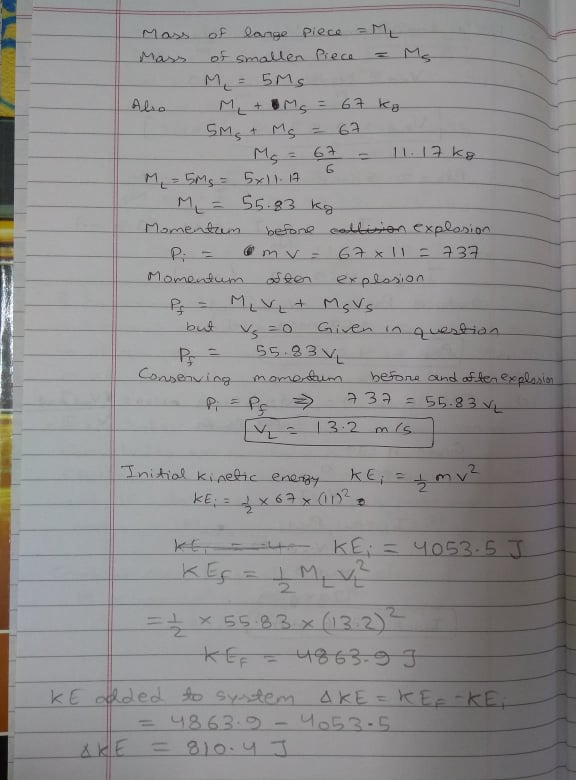Sorry for using pencil my pen stopped working suddenly.

Please rate the answer if you like it and write in comment if you have any doubt

#### Earn Coins

Coins can be redeemed for fabulous gifts.

Similar Homework Help Questions
• ### An object, with mass 42 kg and speed 11 m/s relative to an observer, explodes into...

An object, with mass 42 kg and speed 11 m/s relative to an observer, explodes into two pieces, one 5 times as massive as the other; the explosion takes place in deep space. The less massive piece stops relative to the observer. How much kinetic energy is added to the system during the explosion, as measured in the observer's reference frame?

• ### An object, with mass 93 kg and speed 23 m/s relative to an observer, explodes into...

An object, with mass 93 kg and speed 23 m/s relative to an observer, explodes into two pieces, one 3 times as massive as the other; the explosion takes place in deep space. The less massive piece stops relative to the observer. How much kinetic energy is added to the system during the explosion, as measured in the observer's reference frame

• ### Question 3 An object, with mass 79 kg and speed 24 m/s relative to an observer,...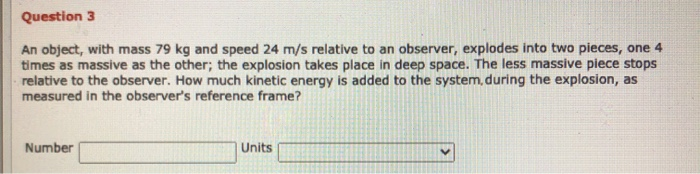Question 3 An object, with mass 79 kg and speed 24 m/s relative to an observer, explodes into two pieces, one 4 times as massive as the other; the explosion takes place in deep space. The less massive piece stops relative to the observer. How much kinetic energy is added to the system, during the explosion, as measured in the observer's reference frame? Number Units

• ### x Incorrect. An object, with mass m and speed vi relative to an observer, explodes into...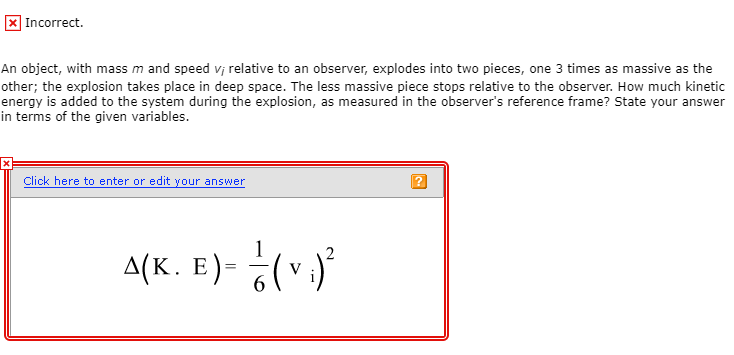x Incorrect. An object, with mass m and speed vi relative to an observer, explodes into two pieces, one 3 times as massive as the other; the explosion takes place in deep space. The less massive piece stops relative to the observer. How much kinetic energy is added to the system during the explosion, as measured in the observer's reference frame? State your answer in terms of the given variables. Click here to enter or edit your answer 4(K. E)-6...

• ### An object with total mass mtotal = 6.4 kg is sitting at rest when it explodes...

An object with total mass mtotal = 6.4 kg is sitting at rest when it explodes into two pieces. The two pieces, after the explosion, have masses of m and 3m. During the explosion, the pieces are given a total energy of E = 45 J. What is the speed of the smaller piece after the collision? What is the speed of the larger piece after the collision? If the explosion lasted for a time t = 0.029 s, what...

• ### 1 object initially at rest explodes into three picces. Piece A has a mass of 5...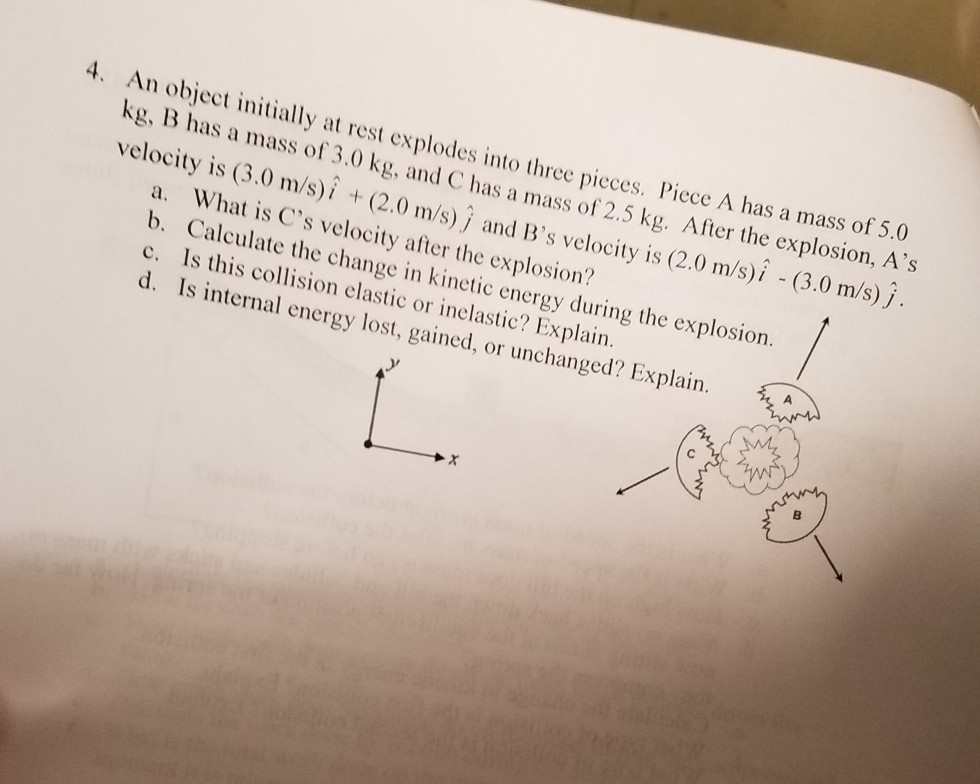1 object initially at rest explodes into three picces. Piece A has a mass of 5 kg, B has a mass of 3.0 kg, and C has a mass of 2.5 kg. After the explosion, A's velocity is (3.0 m/s)i + (2.0 m/s) j and B's velocity is (2.0 m/s)i - (3.0 m/s) j y at rest explodes into three pieces. Piece A has a mass of 5.0 a. What is C's velocity after the explosion? b. Calculate the change...

• ### A particle of mass 1.67Ý10-27 kg moves with a speed of 2.6Ý108 m/s relative to an...

A particle of mass 1.67Ý10-27 kg moves with a speed of 2.6Ý108 m/s relative to an observer. What is the kinetic energy of the particle in the rest frame of the observer?

• ### An object of mass m, initially moving at 6.44 along the x-axis, explodes into two pieces....

An object of mass m, initially moving at 6.44 along the x-axis, explodes into two pieces. The first piece, 83.6 percent of the mass, moves along the negative x-axis at 7.63 m/s. Find the speed, in m/s, of the other piece right after the explosion.

• ### An object with total mass mtotal = 16.1 kg is sitting at rest when it explodes...

An object with total mass mtotal = 16.1 kg is sitting at rest when it explodes into three pieces. One piece with mass m1 = 4.6 kg moves up and to the left at an angle of θ1 = 22° above the –x axis with a speed of v1 = 25 m/s. A second piece with mass m2 = 5.1 kg moves down and to the right an angle of θ2 = 27° to the right of the -y axis...

• ### (3) A particle of mass 3 kg travels along the y-axis with a speed of 5...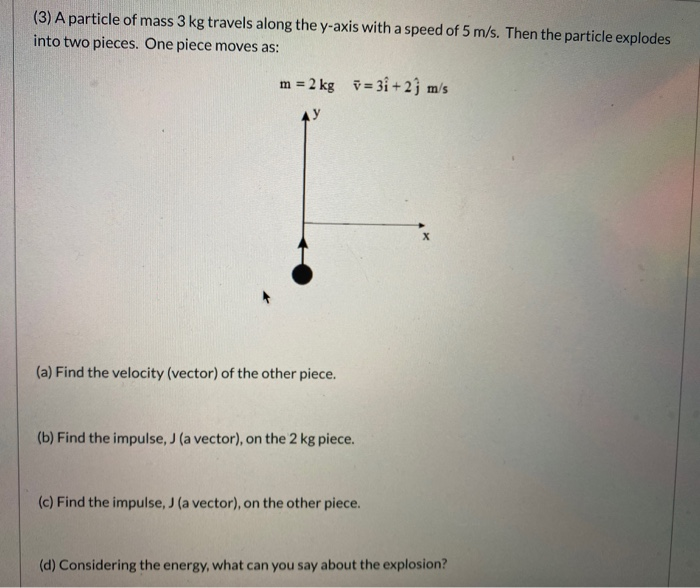(3) A particle of mass 3 kg travels along the y-axis with a speed of 5 m/s. Then the particle explodes into two pieces. One piece moves as: m = 2 kg = 3i+2j m/s (a) Find the velocity (vector) of the other piece. (b) Find the impulse, J (a vector), on the 2 kg piece. (c) Find the impulse, J (a vector), on the other piece. (d) Considering the energy, what can you say about the explosion?